# Stopping rule

(diff) ← Older revision | Latest revision (diff) | Newer revision → (diff)

Letdenote a fixed (continuous) linear functional on. For the numerical approximation ofmost often so-called quadrature formulas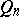are used (cf. also Quadrature formula). These are linear functionals of type(a1)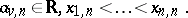The numbers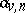are called the weights of, while the numbers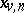are the so-called nodes of. The remainder term of the quadrature formulais the linear functionaldefined by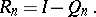(a2)

In order to stop a procedure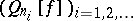, in practice one has to decide whether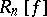is less than a prescribed tolerance or not. Most of the numerical software packages compute exit criteria using functionals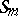of the same type as, i.e.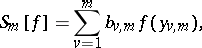(a3)where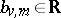,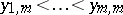, and where the second condition in (a3) is given with regard to the computational complexity of the procedure (cf. also Algorithm, computational complexity of an). Of course, one "hopes" that forone has the inequality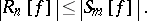(a4)

Since the middle of the 1960s, many new and sophisticated algorithms for the numerical approximation of functionals, in particular for numerical integration (cf. Integration, numerical), have been developed. See [a2], [a4], [a11], [a13] and [a3], [a5], [a9]. Most of these automatic algorithms use one or several estimates for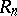of the type (a4), which often are obtained by the help of a further quadrature formula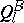: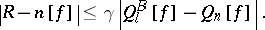(a5)

Here the values ofare determined by asymptotic properties of, respectively, as well as by numerical experience (cf. e.g. [a1], [a7], [a10]). For the latter, different testing techniques are used, see e.g. [a4], [a6], [a12], [a13]. Naturally, a mathematical proof that such estimates (a4), respectively (a5), "almost" hold is not realistic. However, mathematical results describing special classes of functions and special functionals, for which such inequalities are valid, may give some hints for practical application.

In particular, let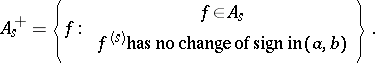A functionalof type (a3) is called a Peano stopping functional for the quadrature formulaif (a4) holds for every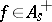. There are several results for this type of stopping functionals which are based on Peano kernel theory; for a survey see [a8].

How to Cite This Entry:
Stopping rule. Encyclopedia of Mathematics. URL: http://encyclopediaofmath.org/index.php?title=Stopping_rule&oldid=15510
This article was adapted from an original article by K.-J. FÃ¶rster (originator), which appeared in Encyclopedia of Mathematics - ISBN 1402006098. See original article